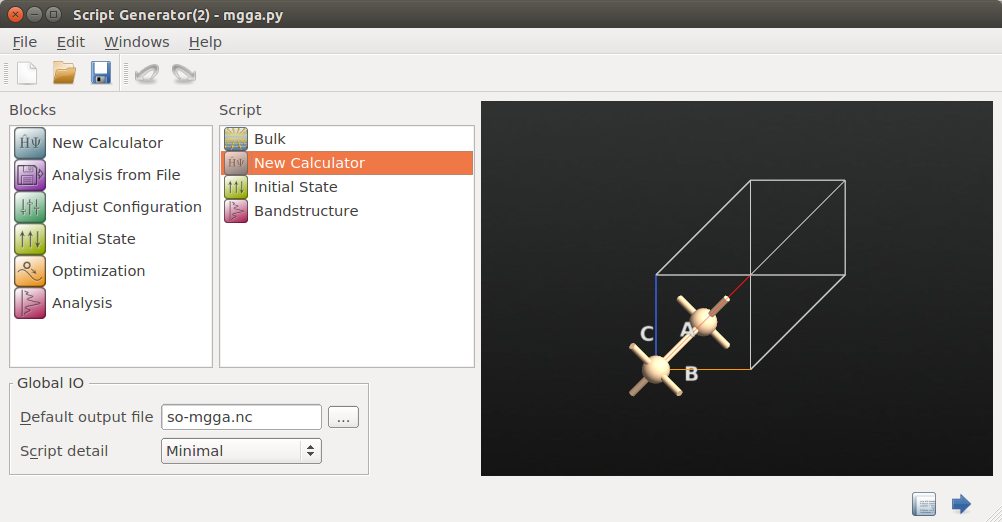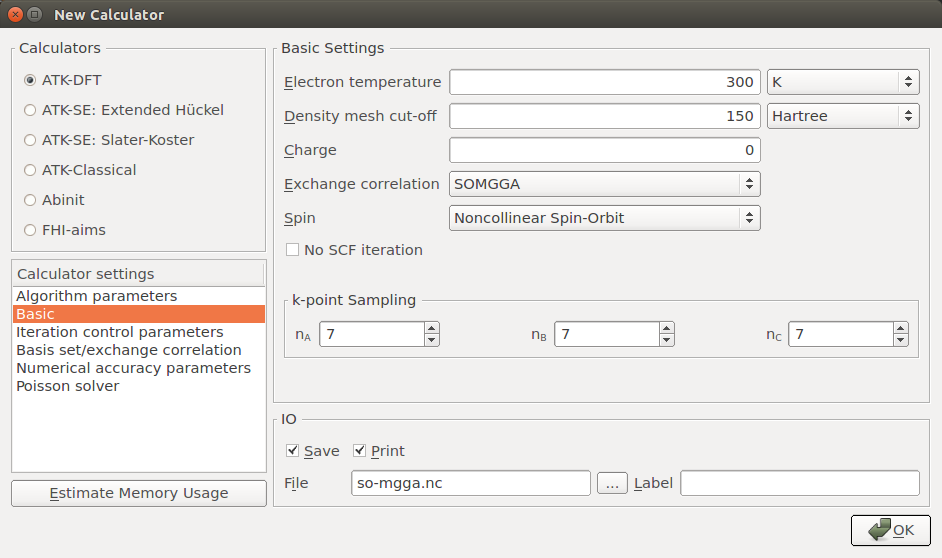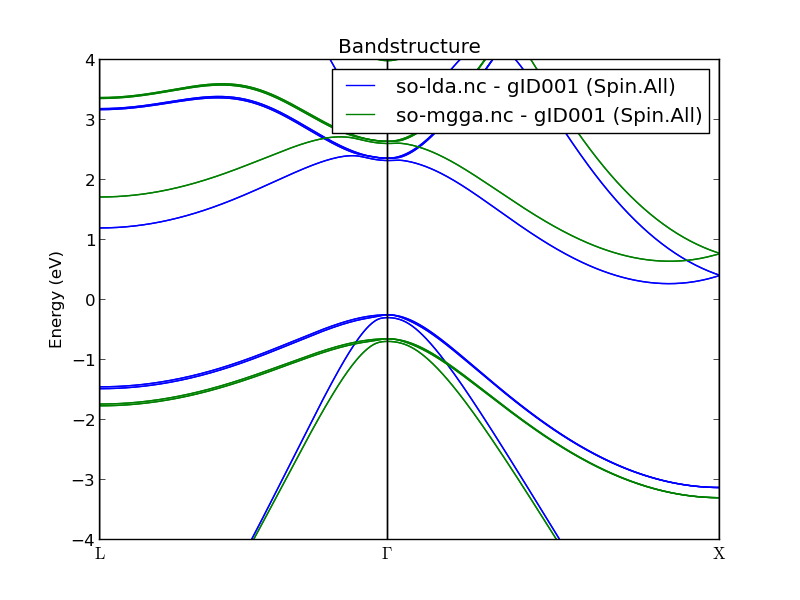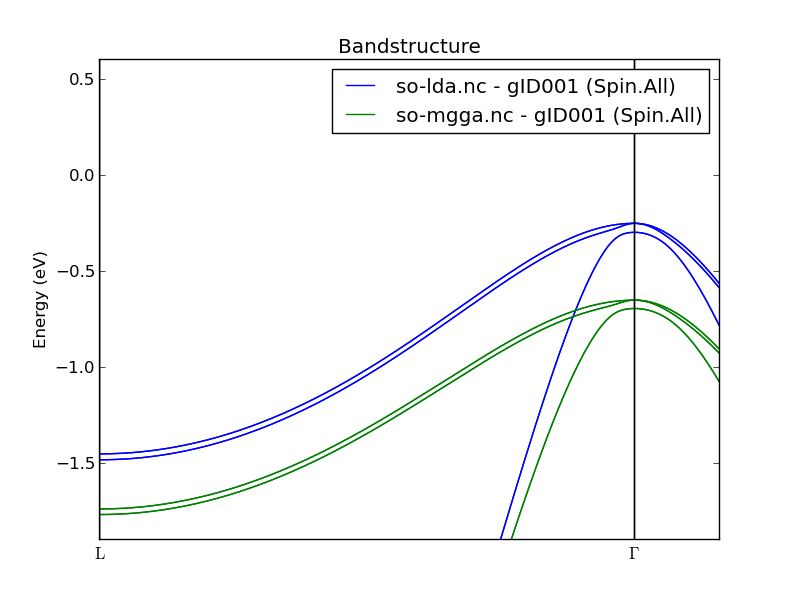# Silicon bandstructure and DOS using QuantumATK and Quantum ESPRESSO¶

In this tutorial you will learn how to use QuantumATK and Quantum ESPRESSO for calculating and analyzing the bandstructure and density of states of bulk silicon.

Outline: We first use QuantumATK to create Quantum ESPRESSO (QE) input files for bandstructure and density of states (DOS) calculations. We then run the calculations and use QuantumATK to visualize and analyze the results.

Many more video tutorials are available on the QuantumWise YouTube channel.

Note

This tutorial uses scripts that were prepared in another tutorial: QuantumATK as GUI for Quantum ESPRESSO. If you have not done so, please have a look at that tutorial before proceeding with this one.

## Bandstructure¶

The ground state electron density from a Quantum ESPRESSO calculation is easily reused for mapping out the bandstructure along lines in reciprocal space. We will therefore reuse the input and output from the tutorial QuantumATK as GUI for Quantum ESPRESSO.

### Creating the input file¶

• Navigate to the directory containing those files, and copy “scf.in” to “bs.in”.

Only a few changes to the QE input file are needed:

1. Open “bs.in” in your favorite editor.
2. In the CONTROL section:
• change calculation from scf to bands.
• change the verbosity option from low to high.
1. Now instruct QE to map out the W-G-X-W-L-G route in k-space by modifying the K_POINTS card:
• Change {automatic} to {crystal_b}.

• Remove all the remaining lines and insert the following ones instead:

6
0.50  0.25  0.75  30 !W
0.00  0.00  0.00  30 !G
0.50  0.00  0.50  30 !X
0.50  0.25  0.75  30 !W
0.50  0.50  0.50  30 !L
0.00  0.00  0.00  30 !G


This will generate 5 route segments with 30 points each.

1. Save the “bs.in” input file. It should now look like illustrated below.
&CONTROL
calculation='bands',
outdir='silicon',
prefix='calc',
pseudo_dir='.',
verbosity='high',
tstress=.false.,
tprnfor=.false.,
/

&SYSTEM
ibrav=2,
celldm(1)=10.2623466921d0,
nat=2,
ntyp=1,
ecutwfc=30.0d0,
ecutrho=300.0d0,
nbnd=10,
input_dft='PBE',
/

&ELECTRONS
diagonalization='david',
conv_thr=1d-08,
mixing_mode='plain',
mixing_beta=0.700d0,
/

ATOMIC_SPECIES
Si 28.085500d0 Si.pbe-n-rrkjus_psl.0.1.UPF

ATOMIC_POSITIONS {alat}
Si   0.0000000000d0   0.0000000000d0   0.0000000000d0
Si   0.2500000000d0   0.2500000000d0   0.2500000000d0

K_POINTS {crystal_b}
6
0.50  0.25  0.75  30 !W
0.00  0.00  0.00  30 !G
0.50  0.00  0.50  30 !X
0.50  0.25  0.75  30 !W
0.50  0.50  0.50  30 !L
0.00  0.00  0.00  30 !G


### Running the calculation¶

Run the bandstructure calculation and send the standard output to bs.out, which is the file from which QuantumATK will read the bandstructure:

pw.x < bs.in > bs.out


### Plotting and analyzing the results¶

The QuantumATK LabFloor now contains a bandstructure object, as highlighted in the image below.You can use the Bandstructure Analyzer… plugin to visualize the silicon bandstructure and easily measure electronic band gaps:

1. Highlight the bandstructure object, and click on Bandstructure Analyzer in the right panel.

2. A plot of the bandstructure pops up, and you can use the mouse to investigate the bands and individual k-points.

3. Holding down the left mouse botton, you can measure band energy differences and the corresponding k-vector displacements.You can also export the bandstructure as plain text for plotting with other tools:

1. Make sure bandstructure object in the LabFloor is highlighted, then click the Export plugin.

2. Choose a filename and save the text-file.## Density of states¶

Let us finally compute the silicon DOS. We first sample the ground state electronic eigenvalues on a very fine k-point grid, and then use the QE executable dos.x to compute the total DOS.

### Input files¶

1. Copy the the input file “scf.in” to “nscf.in”, and apply a few changes:

• Change the calculation type to nscf. This will invoke a non-selfconsistent calculation.
• Add the line occupations=’tetrahedra’, to the SYSTEM cards.
• Increase the size of the k-point grid to 32x32x32.
2. The input file “nscf.in” should now look like this:

&CONTROL
calculation='nscf',
outdir='silicon',
prefix='calc',
pseudo_dir='.',
verbosity='low',
tstress=.false.,
tprnfor=.false.,
/

&SYSTEM
ibrav=2,
celldm(1)=10.2623466921d0,
nat=2,
ntyp=1,
ecutwfc=30.0d0,
ecutrho=300.0d0,
nbnd=10,
input_dft='PBE',
occupations='tetrahedra',
/

&ELECTRONS
diagonalization='david',
conv_thr=1d-08,
mixing_mode='plain',
mixing_beta=0.700d0,
/

ATOMIC_SPECIES
Si 28.085500d0 Si.pbe-n-rrkjus_psl.0.1.UPF

ATOMIC_POSITIONS {alat}
Si   0.0000000000d0   0.0000000000d0   0.0000000000d0
Si   0.2500000000d0   0.2500000000d0   0.2500000000d0

K_POINTS {automatic}
32 32 32 0 0 0

3. We also need an input file for the actual DOS calculation. Use the following and save it as “dos.in”:

&DOS
outdir='silicon',
prefix='calc',
Emin=-10,
Emax=20,
DeltaE=0.05,
fildos='silicon.dos',
/


Note

Please note that the energy window, defined by Emin and Emax, spans approximately 15 eV on both sides of the Fermi level (6.5 eV in this case).

Warning

The DOS is written to the file identified by fildos. It is very important that it is named the same as outdir plus the .dos extension. Otherwise, QuantumATK will not be able to properly identify the file as a QE DOS file.

### Running the calculations¶

Finally, we can run the calculations.

1. Run the non-selfconsistent calculation and send the standard output to nscf.out:
pw.x < nscf.in > nscf.out


This gives us a well-resolved Kohn-Sham eigenvalue spectrum corresponding to the ground state electron density.

1. Run the DOS calculation by invoking the dos.x executable:
dos.x < dos.in > dos.out


### Plotting and analyzing the results¶

1. The QuantumATK LabFloor now also contains a density of states object, as highlighted in the image below.2. Click on 2D Plot in the plugin panel to plot the DOS. It should look like illustrated below.We see the well-known silicon density of states with a band gap of roughly 0.6 eV.